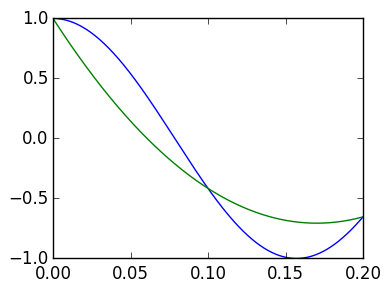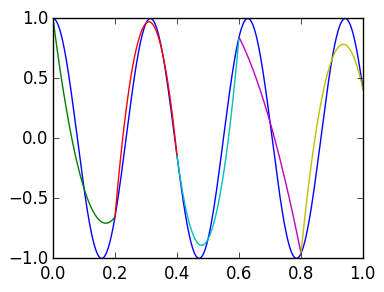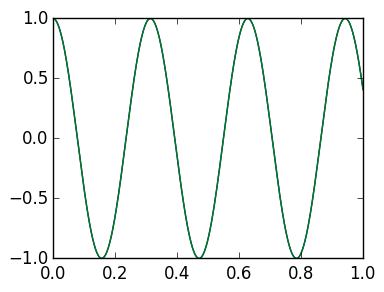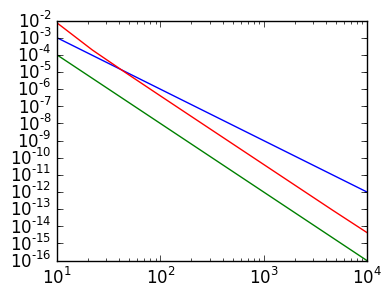# Lab 9: Simpson's Rule¶

In lectures we saw that, for non-periodic functions, that the right-hand rule had $O(n^{-1})$ accuracy and the trapezium rule had $O(n^{-2})$ accuracy. The right-hand rule used a constant approximation to the integrand in each panel, while the trapezium rule used an affine approximation.

In this lab, we explore using a quadratic approximation. We will see that not only do we beat $O(n^{-2})$ accuracy, but we beat it more than expected.

Warning One should never use any of these methods for non-periodic smooth functions, as much better alternatives exist, that also have superalgebraic convergence, like trapezium rule applied to periodic functions. While Simpson's rule is commonly taught, I'm not sure it's ever useful in practice.

The first step is to construct a quadratic approximation that matches a function at $x_0 = 0,x_1 = h$ and $x_2 = 2h$, where $x_k \triangleq kh$.

Exercise 1(a) What polynomial interpolates $f(x)$ at $x_0,x_1,x_2$? (Hint: one can either use a Vandermonde matrix, or construct it directly.) Plot the function $\cos(20x)$ versus its quadratic interpolation for $h=0.1$ in $x \in [0,2h]$

In :
using PyPlot

f=x->cos(20x)

g=linspace(0.,2h,1000)

h=0.1

p=x->f(0.)*(x-h).*(x-2h)/(2h^2) + f(h)*x.*(x-2h)/(-h^2) + f(2h)*x.*(x-h)/(2h^2)

plot(g,f(g))
plot(g,p(g));Exercise 1(b) What polynomial interpolates $f(x)$ at $x_k,x_{k+1},x_{k+2}$? Write a function quadinterp(f::Function,k::Integer,h::Float64,x) that returns the interpolating polynomial evaluated at x, where x can be a Float64 or Vector{Float64}. (Hint: use .* to support Vector and Float64 at the same time.)

In :
function quadinterp(f::Function,k::Integer,h::Float64,x)
f(k*h)*(x-(k+1)*h).*(x-(k+2)*h)/(2h^2) + f((k+1)*h)*(x-k*h).*(x-(k+2)h)/(-h^2)  +
f((k+2)*h)*(x-k*h).*(x-(k+1)*h)/(2h^2)
end

Out:
quadinterp (generic function with 3 methods)

Exercise 1(c) Use quadinterp to plot the piecewise polynomial approximation, by calling it with $k=0,2,4,\ldots,n-2$ where $n$ is even and $h = 1/n$. Make sure to use a plotting grid between $x_k$ and $x_{k+2}$ for each piece.

In :
f=x->cos(20x)

g=linspace(0.,1.,1000)

plot(g,f(g))

n=10
h=1/n

for k=0:2:n-2
g=linspace(k*h,(k+2)*h,100)
endExercise 1(d) Create a function pwquadinterp(f::Function,n::Integer) that, for an even n, returns an anonymous function that is the piecewise quadratic interpolation to f with h=1/n. (Hint: use

x->begin
## Do stuff and return value
end


to write a multiline anonymous function.)

In :
function pwquadinterp(f::Function,n::Integer)
h=1/n
x->begin
for k=0:2:n-2
if k*h≤x≤(k+2)*h
end
end
return 0.
end
end

Out:
pwquadinterp (generic function with 1 method)

Exercse 1(e) Show that the plot is the same as before for n=10. Increase n to ensure that the plot converges. (Hint: use

g=linspace(0.,1.,1000)
v=map(p,g)


to evaluate p at the grid if pwquadinterp doesn't work for Vector inputs.)

In :
p=pwquadinterp(f,100)

g=linspace(0.,1.,1000)

plot(g,f(g))
plot(g,map(p,g));## Simpson's rule¶

The preceding constructed the piecewise quadratic approximation to f. We now want to construct the corresponding quadrature rule.

Exercise 2(a) What is the exact integral

$$\int_0^{2h} p(x) dx$$

where $p(x)$ is the quadratic interpolation of $f(x)$ at $0,h,2h$? Compare your formula with quadgks result applied to your quadinterp function via

quadgk(x->quadinterp(f,0,h,x),0,2h)


and the exact integral

quadgk(f,0,2h)

In :
n=10;h=1/n
h/3*(f(0)+4*f(h)+f(2h))

Out:
-0.04394103223507272
In :
quadgk(x->quadinterp(f,0,h,x),0,2h)

Out:
-0.04394103223507272

Exercise 2(b) If we integrate the quadratic approximation within each panel exactly, what are the $w_k$ so that

$$\int_{0}^1 f(x) dx \approx \sum_{k=0}^n w_k f(x_k)?$$

Exercise 2(c) Write a function simpson(f::Function,n::Integer) that calculates the Simpson's rule approximation to the integral of f. You can assume that $n$ is even. (Hint: Look up Simpson's rule on Wikipedia if you didn't complete 2(b).)

In :
function simpson(f::Function,n::Integer)
h=1/n
ret=f(0.)
for k=2:2:n-2
ret+=2*f(k*h)
end
for k=1:2:n-1
ret+=4*f(k*h)
end

ret+=f(1.)
ret*h/3
end

Out:
simpson (generic function with 1 method)

## Simpson's rule error¶

Exercise 3(a) Ensure that the following returns

4.0769375252158735e-7

In :
simpson(f,100)-quadgk(f,0.,1.)

Out:
4.0769375252158735e-7

Exercise 3(b) Write a function evenlogspace(start,stop,n) that returns logspace(start,stop,n) rounded to a nearby even integer. (Hint: use round(Int,x) to round an integer to the value x. Think about dividing by two.)

In :
function evenlogspace(start,stop,n)
2round(Int,logspace(start,stop,n)/2)
end

Out:
evenlogspace (generic function with 1 method)

Exercise 3(c) Do a loglog plot of the error of Simpson's rule. Compare with plots of $cn^{-\alpha}$ to conjecture the choice of $\alpha$ that gives the convergence. (Hint: this should be a surprise!)

In :
ns=evenlogspace(1,4,10)

err=Float64[abs(ex-simpson(f,n)) for n in ns]

plot(ns,(1.0ns).^(-3))
plot(ns,(1.0ns).^(-4))
loglog(ns,err)Out:
1-element Array{Any,1}:
PyObject <matplotlib.lines.Line2D object at 0x31810ed50>

Exercise 3(d) Advanced Prove the convergence rate of Simpson's rule.